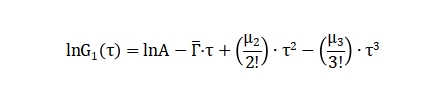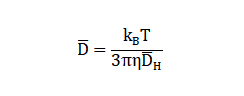Hmoe > Learn > Wiki > What is Z-average and polydispersity index (PDI)?

# What is Z-average and polydispersity index (PDI)?

2023-07-14WIKI

Z-average is the intensity-weighted mean hydrodynamic size of the ensemble collection of particles measured by DLS.

The Z-average is a hydrodynamic size that is determined by an ISO method, i.e., the cumulants method. For the cumulants method, the correlation function is solved by the following expression:where A is the intercept of the correlation function, which represents the signal-noise ratio and Γ  is the average decay rate, which can be substituted into the equation Γ =q2D  to calculate the average diffusion coefficient  D of all particles.  D  is then put into the Stokes-Einstein equation to produce the average particle size DH  , namely the Z-average mean hydrodynamic diameter.For dynamic light scattering, the Z-average is used to present the average particle size, which is the intensity-weighted average size. PDI (Polydispersity Index), defined by PDI=µ2/Γ  , which indicates the width of the distribution.

As a rule of thumb, the following PDIs are shown for different samples.

 Sample Type PDI Mono-dispersed Sample 0 - 0.05 Narrow-dispersed Sample 0.05 – 0.08 Moderate-dispersed Sample 0.08 – 0.7 Wide-dispersed Sample > 0.7

DLS is a technique suitable for samples whose particle size distributions are relatively narrow. For samples with PDI greater than 0.7, DLS measurement might not be applicable.Struggling to grasp particle analysis concepts? Our new Beginner's Guide to Particle Analysis is here to help you grasp these concepts in the most accessible manner possible. Download it as a PDF to read on the train, on the plane, or wherever else there’s no internet.